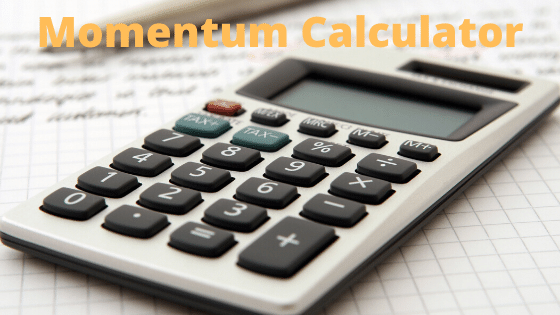# Momentum CalculatorNote
• Enter the values of the two known variables in the text boxes
• Leave the text box empty for the variable you want to solve for
• Click on the calculate button.

Formula used
$p=mv$

## What is momentum

The momentum, p of an object is defined as the product of its mass, m and velocity, v
$p=mv$
Where
p -> Momentum
m -> Mass of the object
v -> Velocity of the object
Momentum has both direction and magnitude so it is a vector quantity. Its direction is the same as that of velocity
The SI unit of momentum is kilogram-meter per second
Example of Few questions where you can use this Momentum Calculator
Question 1
A Body of mass 4 kg is moving with the velocity 10 m/s.Calculate the momentum of the body
Solution
Given m=5 kg, v=10 m/s, p=?
Now Momentum is defined by the formula
$p=mv = 5 \times 10 = 50 \ kg-m/s$

Question 2
A body of mass 2 kg has momentum of 10 kgm/s .Find the velocity of the body.
Solution
Given m=2 kg, v=?, p=10 kgm/s
Now Momentum is defined by the formula
$p=mv$
Rearranging for Velocity
$v= \frac {p}{m} = \frac {10}{2} = 5 \ m/s$

Question 3
A object has momentum and velocity as 75 kgm/s and 10 m/s .Find the mass of the object.
Solution
Given m=?, v=10m/s, p=75 kgm/s
Now Momentum is defined by the formula
$p=mv$
Rearranging for Mass
$m= \frac {p}{v} = \frac {75}{10} = 7.5 \ m$

## How the Linear Momentum Calculator works

1. If mass and velocity given
$p=mv$
2. If mass and momentum are given
$v= \frac {p}{m}$
3. If velocity and Momentum are given
$m = \frac {p}{v}$